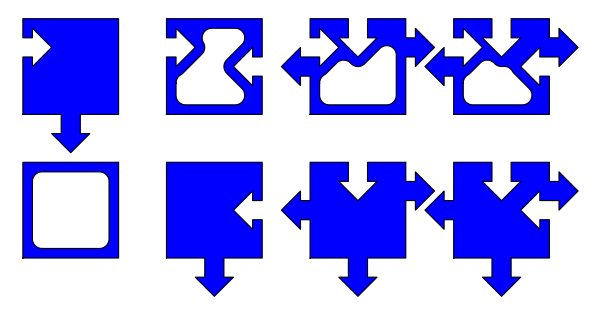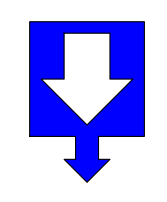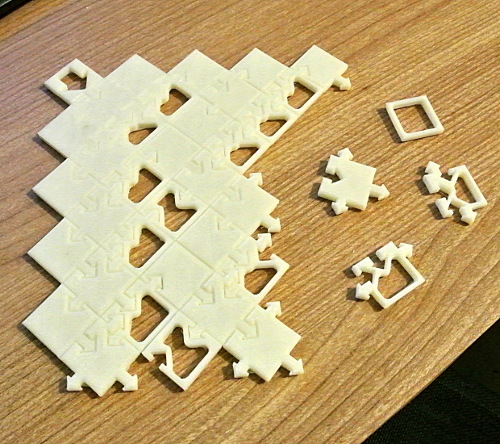Cellular automaton tiles revisited

Previously I wrote about implementing 1D cellular automata as tile puzzles.

My previous solution needed four linker tiles. I've now found a way to get rid of these. Each tile simply transmits the state of the tile above it to its left and right neighbours.

These tiles implement Rule 30:• Filled tiles are "on" and empty tiles are "off".
• Downward arrow on the base transmits the state to the cell below.
• Outward sideways arrows transmit state of N neighbour to E and W neighbours.
• Inward pointing arrows receive state of NW neighbour via W neighbour, state of N neighbour, and state of NE neighbour via E neighbour.

Seed tile:Starting from this seed in the middle of a line of empty squares, the puzzle has a single, infinite solution. The column of tiles below the seed passes tests of randomness used to test pseudo-random number generators. Rule 30 is used as a random number generator in Mathematica. Rule 30 is a simple demonstration of Stephen Wolfram's observation that mathematics is random, and that "creating information" is trivial.

I previously speculated about making these tiles from plastic. Technology to do this is now readily available.Source code:

Requires Python 2, "shapely" python library. Produces files for OpenSCAD in a directory "output". Usage:

python cell_tiles.py 30

You might also like to try other elementary cellular automata, such as Rule 110 which is a computer.

 [æ]